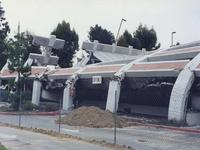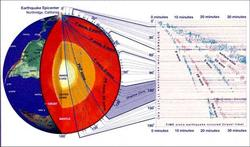# Modeling the interior of the Earth using Seismic Waves

## Summary

This is an inquiry-based student exercise designed to explore how we use seismic waves to understand the structure of the Earth's interior. Students test several models of the interior of the Earth beginning with a simple homogeneous Earth and moving toward more complex multi-layered Earth models. First, students test the viability of a homogeneous (one-layer) Earth using observed P-wave arrival times throughout the globe. Subsequently, the practicality of a two-layer model is explored as students graphically determine ray paths and travel times and perform simple calculations of Snell's law. Finally, students are asked use a publicly available Java program to calculate wave travel times through a model of Earth that they create (with as many or as few layers as they see fit). The ultimate goal involves student generation of a model that reproduces actual measured seismic travel times as closely as possible.

Used this activity? Share your experiences and modifications

## Learning GoalsAt the end of this exercise, students should be able to:
• demonstrate an understanding of reverse modeling (taking measured data and creating a model that produces the measured data)
• explain how models of the Earth's interior are formulated and tested
• use mathematical techniques (graphing, calculation) to discover more about the physical world around us.
At the end of this exercise students may be able to:
• Discuss how modeling might create multiple satisfactory results (non-unique solutions)
• discuss the process of iteration
• examine the process of modeling and errors associated with it, and participate in a discussion of acceptable congruence

## Context for UseI use this lab in both an intro-physical geology class and a non-majors seismology class. The students will have to know about seismic waves beforehand, but there is very little other assumed knowledge. The whole project can take 3-4 hours. The first module, which looks at travel times through a homogenous earth, is useful if you want just a 1 hour or less activity to begin the a discussion of how we use seismic waves to tell what the interior of the Earth is like.

## Description and Teaching Materials

There are several documents you will need for this lab:
Curious how this project came be? Need some diagrams to help explain the concepts? Try this powerpoint presentation (PowerPoint 906kB Feb9 06) and see if it helps.

## Teaching Notes and Tips

For the whole activity I generally use 2 2-hour lab periods. However, because the assignment is open ended, students can take significantly longer. The instructor will need to decide how close they want the student results to be to the actual measured velocities or give them a time limit.

Students will need paper, pencil, protractor, ruler, and a calculator with trigonometric functions. They will also need an internet-connected computer with the current version of Java installed for the third part of this activity.

One common problem with this lab is that the Java program won't run. This is because you don't have the most current version of Java. The applet for module 3 does not run in Java 1.4, but does in 1.5

## Assessment

I grade the submitted pre-lab and lab.

## References and Resources

• The trigonometry page will be helpful for instructors teaching students trig functions.
• A good approximation of the "correct" answer is the PREM (Preliminary Reference Earth Model) by Dziewonski and Anderson (1981 - Phys. Earth. Planet. Int., Vol 25, p.297-356).